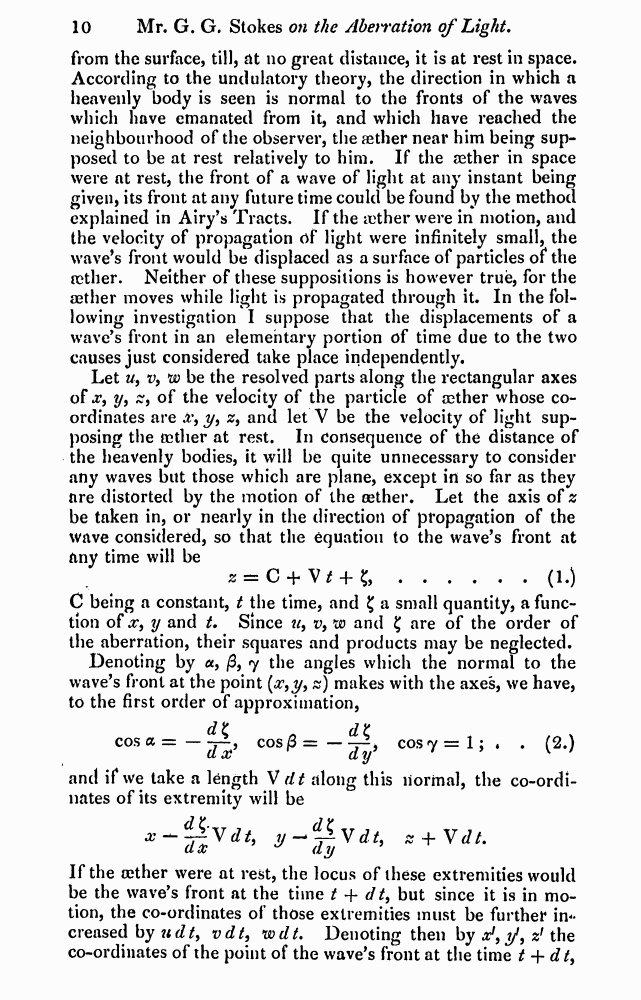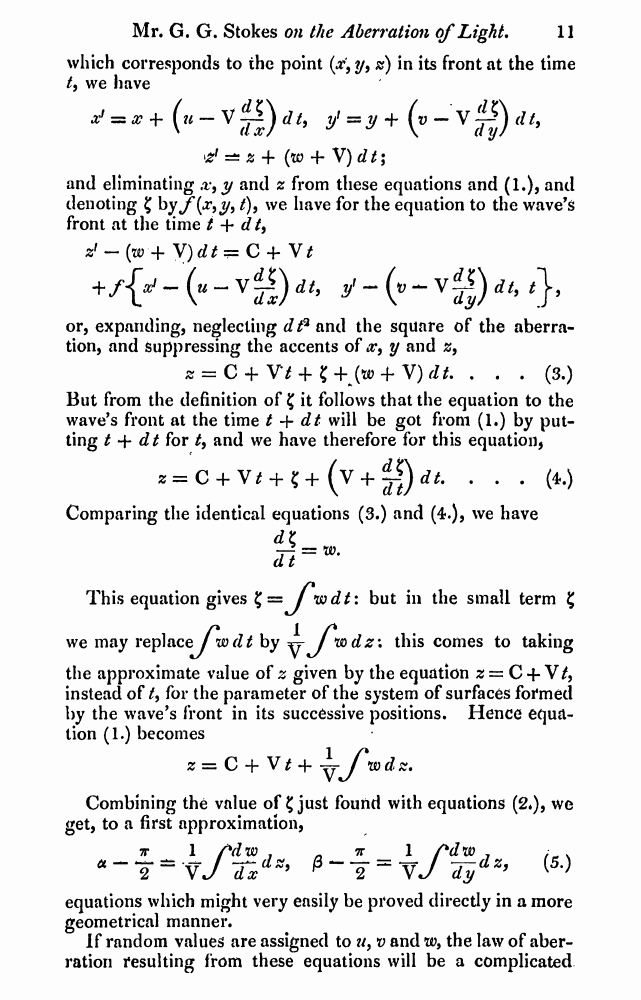﻿ G.G.Stokes "On the Aberration of Light" // Philosophical Magazine 1845, 27: Page 10

# G.G.Stokes "On the Aberration of Light" // Philosophical Magazine 1845, 27: Page 10

В начало   PDF, DjVu   <<<     Страница 10   >>>1  2  9  10 11  12  13  14  15

from the surface, till, at no great distance, it is at rest in space. According to the undulatory theory, the direction in which a heavenly body is seen is normal to the fronts of the waves which have emanated from it, and which have reached the neighbourhood of the observer, the aether near him being supposed to be at rest relatively to him. If the aether in space were at rest, the front of a wave of light at any instant being given, its front at any future time could be found by the method explained in Airy’s Tracts. If the ether were in motion, and the velocity of propagation of light were infinitely small, the wave’s front would be displaced as a surface of particles of the aether. Neither of these suppositions is however true, for the aether moves while light is propagated through it. In the following investigation I suppose that the displacements of a wave’s front in an elementary portion of time due to the two causes just considered take place independently.

Let w, v, w be the resolved parts along the rectangular axes of jt, y9 s, of the velocity of the particle of aether whose coordinates are £*, y, z9 and let V be the velocity of light supposing the tether at rest. In consequence of the distance of the heavenly bodies, it will be quite unnecessary to consider any waves but those which are plane, except in so far as they are distorted by the motion of the aether. Let the axis of z be taken in, or nearly in the direction of propagation of the wave considered, so that the equation to the wave’s front at any time will be

2 = C + ......(I*)

C being a constant, t the time, and £ a small quantity, a function of x, y and t. Since «, v, w and £ are of the order of the aberration, their squares and products may be neglected.

Denoting by a, /3, 7 the angles which the normal to the wave’s front at the point (#,y, z) makes with the axes, we have, to the first order of approximation,

dt » d£ cosa=~dl, cos^=~Tyi cos'y = 1;‘ • (2*)

and if we take a length V dt along this normal, the co-ordi-nates of its extremity will be

If the aether were at rest, the locus of these extremities would be the wave’s front at the time t + dt, but since it is in motion, the co-ordinates of those extremities must be further increased by udt> vdt, wdt. Denoting then by ^,y, z1 the co-ordinates of the point of the wave’s front at the time t + dt9

which corresponds to the point (x’9 y, z) in its front at the time t9 we have

*? = x+(u-V&)dt, y=jr+(B-v^)rf/,

d =± z + (to + V) d t;

and eliminating ,v9 y and z from these equations and (1.), and denoting £ hyf(x9 y9t)9 we have for the equation to the wave’s front at the time t + dt9

zf — {w + V)dt=C + V/

## +/{/ - (» - vg) it, y - (»- vg) i/, <},

or, expanding, neglecting dt3 and the square of the aberration, and Suppressing the accents of y and z9

z = C + Vt + 1'+^w + V)dt. . . . (3.)

But from the definition of £ it follows that the equation to the wave’s front at the time t + dt will be got from (1.) by putting t 4- dt for t9 and we have therefore for this equation,

z=C + V* + ?+ (V + ifydt. . . . (4.) Comparing the identical equations (3.) and (4.), we have

### <*K

77 = w-d t

This equation gives £= J”wdt: but in the small term £

we may replaceylwrZrf by ^ J*mdz\ this comes to taking

the approximate value of z given by the equation z = C + V/, instead of t9 for the parameter of the system of surfaces formed by the wave’s front in its successive positions. Hence equation (1.) becomes

z=C + V^-f—J*wdz.

Combining the value of £ just found with equations (2*), we get, to a first approximation,

7r 1 rdtxi J 7T 1 /*dw J : s

## * 2 ~ vJ dx * P 2 “ VJ dy ’ ^

equations which might very easily be proved directly in a more geometrical manner.

If random values are assigned to a9 v and w9 the law of aberration resulting from these equations will be a complicated